Related Articles
How to utilize time series in Pandas?
• Last Updated : 03 Mar, 2021

The pandas library in python provides a standard set of time series tools and data algorithms. By this, we can efficiently work with very large time series and easily slice and dice, aggregate, and resample irregular and fixed frequency time series.

Time series data is an important form of structured data which is used in finance, economics, ecology, etc. Anything that is observed or measured at many points in time forms a time series.

1. Timestamps: These are the specific instants in time
2. Fixed period: This will represent such as the month of May 25 or the full year 1999.

## Modules in DateTime

• date: This module is used to store the calendar in the format of year, month, date.
• time: This module is used to get and display the time in the format of hours, minutes, seconds, and microseconds.
• datetime: This module is used to store both date and time.
• timedelta: This module is used to get the difference between two datetime values.

Below are various examples that depict how to utilize time series in the pandas library:

Example 1: Display the current date and time. In this program, we are going to display current date and time.

## Python3

 `# import datetime module ``# for getting date and time``from` `datetime ``import` `datetime`` ` `# command to display ``# current date and time``datetime.now()`

Output: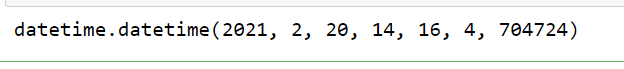Example 2: Program to display hour, minutes, seconds, month, year, day individually from the module.

## Python3

 `# import module``from` `datetime ``import` `datetime`` ` `# display all attributes``a``=``datetime.now()``print``(a.year)``print``(a.day)``print``(a.month)``print``(a.hour)``print``(a.minute)``print``(a.second)``print``(a.microsecond)``print``(a.date)`

Output: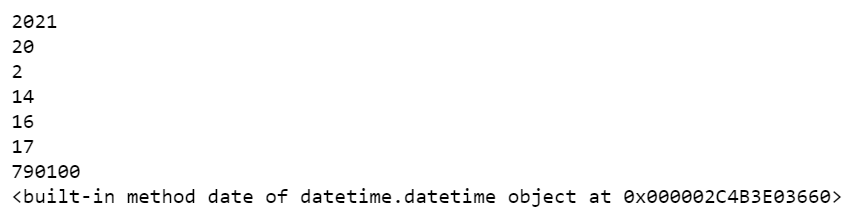Example 3: Difference between two dates. We can get hours, days and minute difference using timedelta module.

## Python3

 `# importing time delta module``from` `datetime ``import` `timedelta`` ` `# subtracting date from year 2027 to 2021``deltaresult ``=` `datetime(``2027``, ``5``, ``7``) ``-` `datetime(``2021``, ``6``, ``24``)`` ` `# display the result``print``(deltaresult)`` ` `# to get days``print``(deltaresult.days)`` ` `# to get seconds difference``print``(deltaresult.seconds)`

Output:If we want to generate time series data python will support date_range module. This will generate dates within the given frequency. It is available in the pandas module.

Syntax: pandas.date_range(start=None, end=None, periods=None, freq=None)

Parameters:

• start: start date time from start date.
• end: specify end date time.
• freq: represents a frequency like hours or minutes or seconds.

Example 4: In this program, we can start with 2021 January 1st and display the dates up to march using the date_range method.

## Python3

 `# importing pandas module``import` `pandas as pd`` ` `# using date_range function to generate ``# dates from january 1 2021 to ``# march 16 2021 as periods = 75``dates ``=` `pd.date_range(``'1/1/2021'``, periods``=``75``)`` ` `print``(dates)`

Output: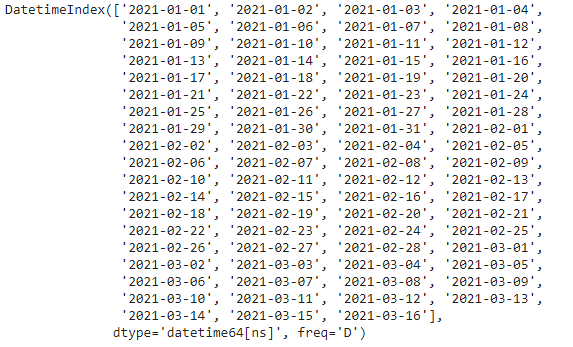Generating values to corresponding dates by using timeseries as indices.

Example 5: In this program, we are giving values to dates by setting dates as indexes to each value.

## Python3

 `# importing pandas module``import` `pandas as pd``# importing numpy module for generating values``import` `numpy as np`` ` `# using date_range function to generate dates``# from january 1 2021 to march 16 2021 as periods = 75``dates ``=` `pd.date_range(``'1/1/2021'``, periods``=``75``)`` ` `# giving values to dates``results ``=` `pd.Series(np.arange(``75``), index``=``dates)`` ` `# print results``print``(results)`` ` `# converting to data frame``print``(pd.DataFrame(results))`

Output: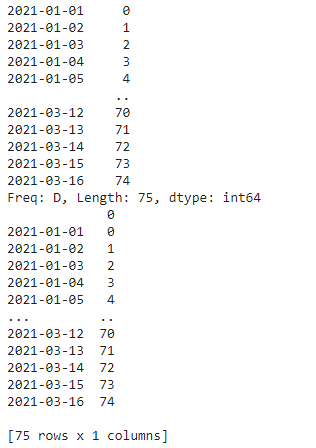We can convert data string columns to datetime type by using the below method.

Syntax:

pandas.to_datetime(column_name)

Example 6: In this program, we will convert string data into datetime type.

## Python3

 `# importing pandas module for data frame``import` `pandas as pd`` ` `# creating data frame for different customers ``# in a shop with respect to dates``data ``=` `pd.DataFrame({``'dates'``: [``'1/2/2021'``,``                               ``'2/4/2020'``,``                               ``'1/3/2021'``,``                               ``'4/12/2017'``],``                     ``'customers'``: [``100``, ``30``, ``56``, ``56``]})`` ` `# display original data``data`` ` `# converting dates column to date ``# using pandas.to_datetime function``data[``'dates'``] ``=` `pd.to_datetime(data[``'dates'``])`` ` `# data after conversion``data`

Output: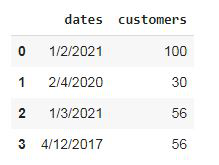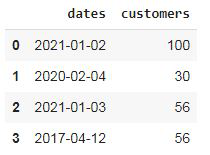Example 7: In this program, we are going to take some time series data as an index, convert it and verify whether they are equal or not.

## Python3

 `# importing pandas module for data frame``import` `pandas as pd`` ` `# creating data frame for different customers ``# in a shop with respect to dates``data ``=` `pd.DataFrame({``'dates'``: [``'1/2/2021'``, ``'2/4/2020'``, ``                               ``'1/3/2021'``, ``'4/12/2017'``,``                               ``'1/2/2021'``, ``'2/4/2020'``, ``                               ``'1/3/2021'``], ``                     ``'customers'``: [``100``, ``30``, ``56``, ``                                   ``56``, ``23``, ``45``, ``67``]})``# display original data``data`` ` `# converting dates column to date ``# using pandas.to_datetime function``data[``'dates'``] ``=` `pd.to_datetime(data[``'dates'``])`` ` `# after conversion``data`` ` `# finding unique time series data``print``(data[``'dates'``].nunique())`` ` `# counting each series data``data[``'dates'``].value_counts()`

Output: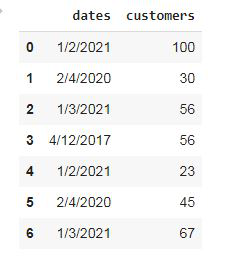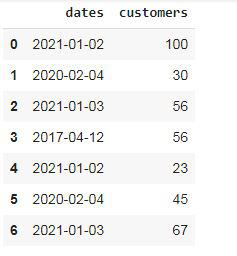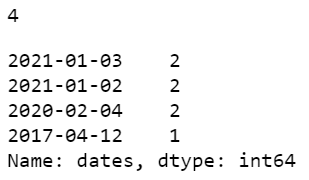Example 8: Program to display a histogram from a data frame having DateTime objects.

## Python3

 `# importing pandas module for data frame``import` `pandas as pd`` ` `# creating data frame for different customers``# in a shop with respect to dates``data ``=` `pd.DataFrame({``'dates'``: [``'1/2/2021'``, ``'2/4/2020'``,``                               ``'1/3/2021'``, ``'4/12/2017'``,``                               ``'1/2/2021'``, ``'2/4/2020'``,``                               ``'1/3/2021'``],``                     ``'customers'``: [``100``, ``30``, ``56``,``                                   ``56``, ``23``, ``45``, ``67``]})``# display original data``data`` ` `# converting dates column to date``# using pandas.to_datetime function``data[``'dates'``] ``=` `pd.to_datetime(data[``'dates'``])`` ` `# depict visualization``data[``'dates'``].hist(figsize``=``(``10``, ``5``), color``=``"green"``)`

Output: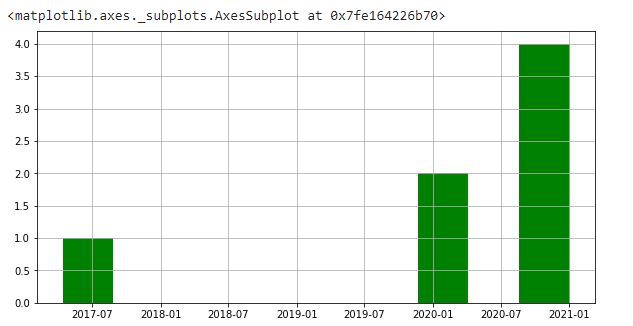Attention geek! Strengthen your foundations with the Python Programming Foundation Course and learn the basics.

To begin with, your interview preparations Enhance your Data Structures concepts with the Python DS Course.

My Personal Notes arrow_drop_up